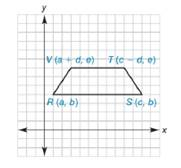Chapter 10.2, Problem 39EElementary Geometry For College St...

7th Edition
Alexander + 2 others
ISBN: 9781337614085

Solutions

Chapter
SectionElementary Geometry For College St...

7th Edition
Alexander + 2 others
ISBN: 9781337614085
Textbook Problem

Show that quadrilateral R S T V is an isosceles trapezoid.To determine

To prove:

The quadrilateral RSTV is an isosceles trapezoid.Explanation

To prove that the quadrilateral is an isosceles trapezoid, we have to prove the following conditions.

Two sides are need to be parallel, so that the quadrilateral is a trapezoid.

For an isosceles trapezoid, the other two sides are equal.

By theorem,

If two lines are parallel, then their slopes are equal.

(i.e) If l1l2, then m1=m2.

The slope of the line that contains the points x1,y1 and x2,y2 is given by

m=y2-y1x2-x1 for x2x1

Let mRS- and mTV- are the slopes of the line RS- and VT- respectively.

The given points are Ra, b, and Sc, b.

Using the slope formula and choosing x1=a, x2=c, y1=b, and y2=b.

mRS-=b-bc-a

mRS-=0c-a

mRS-=0

The given points are Va+d, e and Tc-d, e.

Using the slope formula and choosing x1=a+d, x2=c-d, y1=e, and y2=e.

mVT-=e-ec-d-a-d

mVT-=0c-a-2d

mVT=0

Here mRS-=mVT

Thus RS VT

If slopes are equal, then those lines are parallel

Still sussing out bartleby?

Check out a sample textbook solution.

See a sample solution

The Solution to Your Study Problems

Bartleby provides explanations to thousands of textbook problems written by our experts, many with advanced degrees!

Get Started

Sketch the graph of the equation 3x 4y =24.

Applied Calculus for the Managerial, Life, and Social Sciences: A Brief Approach

Find the derivative. Simplify where possible. 39. g(t)=tcotht2+1

Single Variable Calculus: Early Transcendentals, Volume I

Calculate y'. 49. y=cos(etan3x)

Single Variable Calculus: Early Transcendentals